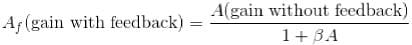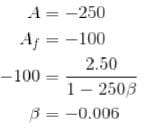Bipolar Junction Transistor NAT Level -1

# Bipolar Junction Transistor NAT Level -1 - Physics

Test Description

## 10 Questions MCQ Test Topic wise Tests for IIT JAM Physics - Bipolar Junction Transistor NAT Level -1

Bipolar Junction Transistor NAT Level -1 for Physics 2023 is part of Topic wise Tests for IIT JAM Physics preparation. The Bipolar Junction Transistor NAT Level -1 questions and answers have been prepared according to the Physics exam syllabus.The Bipolar Junction Transistor NAT Level -1 MCQs are made for Physics 2023 Exam. Find important definitions, questions, notes, meanings, examples, exercises, MCQs and online tests for Bipolar Junction Transistor NAT Level -1 below.
Solutions of Bipolar Junction Transistor NAT Level -1 questions in English are available as part of our Topic wise Tests for IIT JAM Physics for Physics & Bipolar Junction Transistor NAT Level -1 solutions in Hindi for Topic wise Tests for IIT JAM Physics course. Download more important topics, notes, lectures and mock test series for Physics Exam by signing up for free. Attempt Bipolar Junction Transistor NAT Level -1 | 10 questions in 30 minutes | Mock test for Physics preparation | Free important questions MCQ to study Topic wise Tests for IIT JAM Physics for Physics Exam | Download free PDF with solutions
 1 Crore+ students have signed up on EduRev. Have you?
*Answer can only contain numeric values
Bipolar Junction Transistor NAT Level -1 - Question 1

### For a transistor circuit, VCE when Vin = 0V (in volts).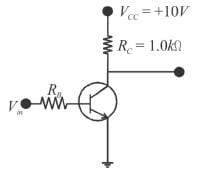Detailed Solution for Bipolar Junction Transistor NAT Level -1 - Question 1

When Vin = 0V, the transistor is in cut-off (acts like an open switch) and VCE =VCC = 10V.

*Answer can only contain numeric values
Bipolar Junction Transistor NAT Level -1 - Question 2

### For a BJT, the current amplification factor ∝ = 0.9 . This transistor is connected in common emitter configurations. When the base current changes by 0.4 mA, the change in collector current will be (in mA).

Detailed Solution for Bipolar Junction Transistor NAT Level -1 - Question 2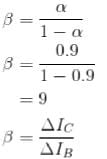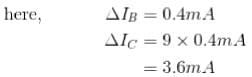*Answer can only contain numeric values
Bipolar Junction Transistor NAT Level -1 - Question 3

### A power amplifier gives 150 W output of or an input of 1.5W. The gain in dB is :

Detailed Solution for Bipolar Junction Transistor NAT Level -1 - Question 3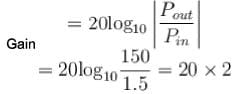= 40 dB

*Answer can only contain numeric values
Bipolar Junction Transistor NAT Level -1 - Question 4

What is the current gain for a common-base configuration where IE = 4.2 mA and IC = 4.0 mA?

Detailed Solution for Bipolar Junction Transistor NAT Level -1 - Question 4

Ie = 4.2ma (Given)

Ic = 4.0ma (Given)

The question follows the common base configuration in the circuit configuration of a bipolar transistor, where the emitter is the input electrode, collector is the output electrode and the base is reference electrode for both input and the output.

Thus,

Current gain = Ic / Ie  =I(out)/I(in)

where, IC=Output Current and IE = Input Current

= 4 / 4.2

= 0.95

Therefore, the current gain for a common-base configuration is 0.95

*Answer can only contain numeric values
Bipolar Junction Transistor NAT Level -1 - Question 5

Figure shows a common emitter amplifier with β = 100. What is the maximum peak to peak input signal (in mV) for which distortion free output may be obtained Assume VBE = 0 r= 20 Ω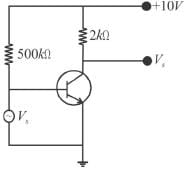Detailed Solution for Bipolar Junction Transistor NAT Level -1 - Question 5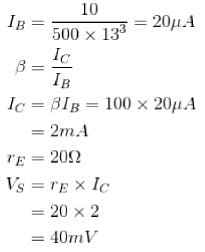But for maximum peak to peak input signal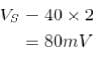*Answer can only contain numeric values
Bipolar Junction Transistor NAT Level -1 - Question 6

In the following circuit, Tr1 and Tr2 are identical transistors having VBE = 0.7V. The current passing through the transistor Tr2 is (in mA).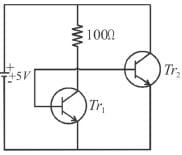Detailed Solution for Bipolar Junction Transistor NAT Level -1 - Question 6

Applying KVL,
5=100x1 + 0.7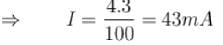*Answer can only contain numeric values
Bipolar Junction Transistor NAT Level -1 - Question 7

If the transistor amplifier has a gain of 20dB, then the ratio of output to input power is :

Detailed Solution for Bipolar Junction Transistor NAT Level -1 - Question 7

The ratio of output to input power is known as power amplification.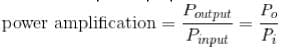The logarithm of the power amplification is represented in bel.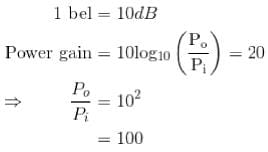*Answer can only contain numeric values
Bipolar Junction Transistor NAT Level -1 - Question 8

A common emitter amplifier has voltage gain of 50, an input impedance of 1000Ω and an output impedance of 200Ω. The power gain of the amplifier will be (in dB).

Detailed Solution for Bipolar Junction Transistor NAT Level -1 - Question 8

Power gain = Voltage gain x current gain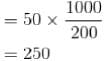*Answer can only contain numeric values
Bipolar Junction Transistor NAT Level -1 - Question 9

The input signal given to a common emitter amplifier having a voltage gain of 20 is Vin = 20 cos (5t + 20°). The corresponding output signal has an amplitude equal to ?

Detailed Solution for Bipolar Junction Transistor NAT Level -1 - Question 9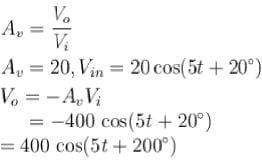*Answer can only contain numeric values
Bipolar Junction Transistor NAT Level -1 - Question 10

The feedback ratio of an amplifier, which an amplification of a negative feedback, changes the voltage gain the voltage gain from - 250 to - 100 is (give magnitude only).

Detailed Solution for Bipolar Junction Transistor NAT Level -1 - Question 10